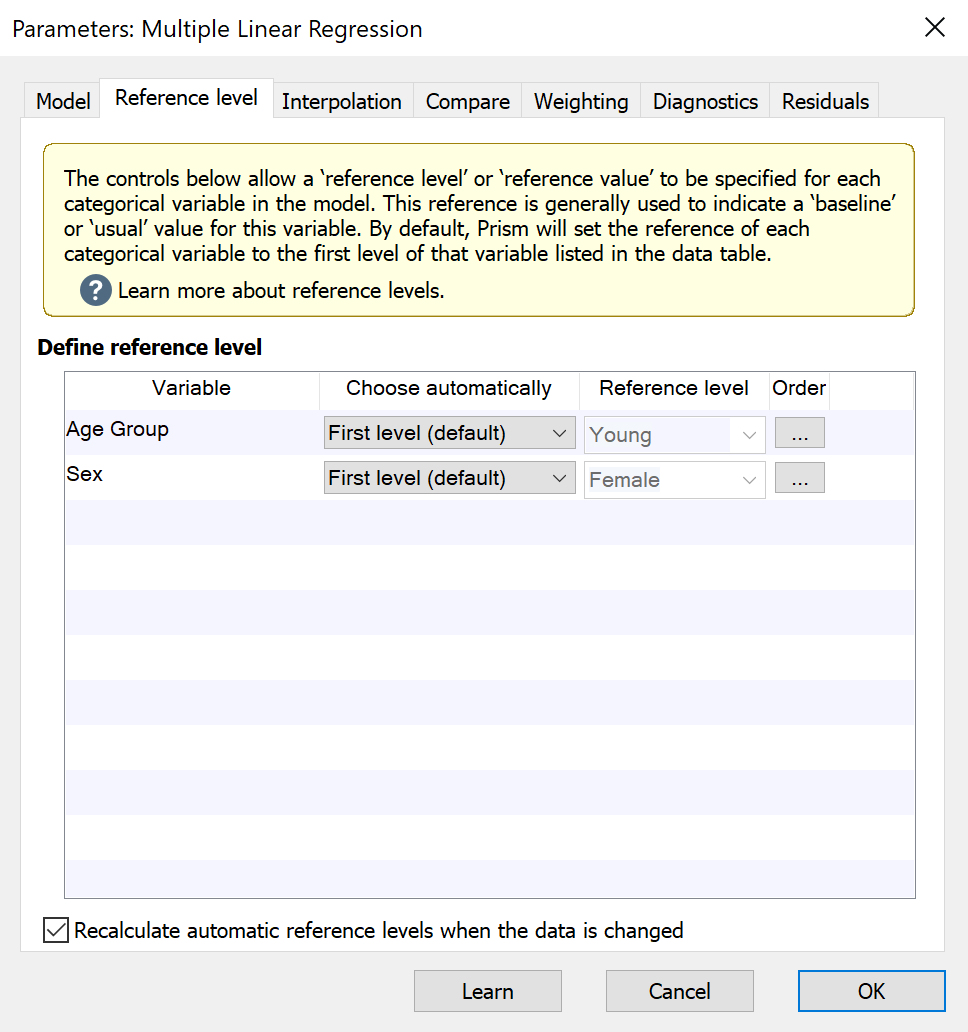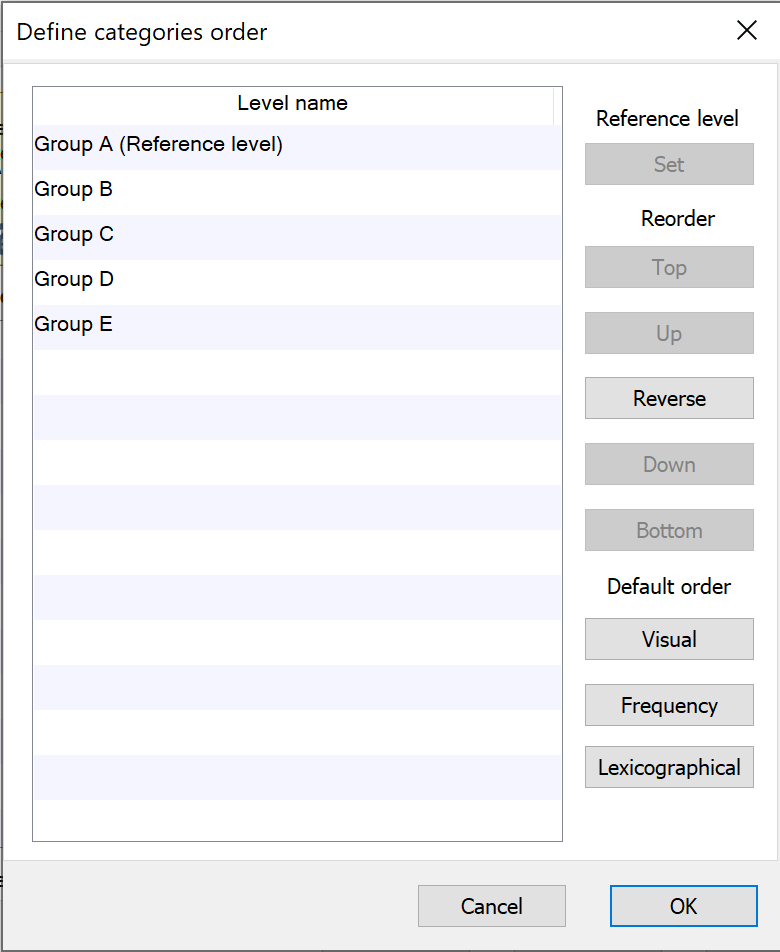## Please enable JavaScript to view this site.

 Setting reference levels for multiple regression

When a categorical variable is included in a regression model as a predictor, Prism automatically encodes this variable using “dummy coding”. This process generates (behind the scenes) a number of new variables equal to the number of levels of the original categorical variable minus one. In other words, if a categorical predictor variable had 5 unique levels (A, B, C, D, and E, for example), dummy coding would generate 4 new variables. If a categorical predictor variable only had two unique levels (Male and Female, for example), dummy coding would generate only one new variable. In this way, every level of a categorical predictor variable except for one gets a new variable that is used in the regression analysis. Additionally, a beta coefficient is calculated for each of these new variables.

But what do those beta coefficients represent? And what about the level that doesn’t get a new variable? These questions both have to do with the concept of a reference level for a categorical predictor variable.

## What are reference levels

The reference level of a categorical predictor variable is often considered the “baseline” or “usual” value that is observed for the given variable. In the process of dummy coding, the variable for the reference level is left out since it would simply contain “0” for every observation. Instead, the reference level is used as a means of interpretation of the generated regression model. Let’s use an example to make this clear:

Consider a model that includes the categorical predictor variable “Sex” with levels “Male” and “Female”. If “Female” is our reference level, then the predicted model will include a beta coefficient for “Male”, but will not include one for “Female”. What the beta coefficient for “Male” in this case tells us is how much the outcome variable is predicted to change between men and women, holding all other variables constant. In other words, if the beta coefficient for “Male” is -5.632, then (holding all other variables constant), the outcome variable is predicted to be 5.632 smaller for males than for females.## How to specify reference levels

On the Reference level tab, each of the categorical predictor variables included in the regression model will be listed under “Define reference level”. For each variable, you can choose to define the reference level automatically or to define a level manually. Prism provides a number of ways to specify a reference level automatically based on the data in the data table. These methods include:

First level (default). This will select the first level of the variable in the data table. Note that if the order of the rows in the data table change, this reference level may also change!

Last level. This will select the final level of the variable in the data table. Note that if the order of the rows in the data table change, this reference level may also change!

Most frequent level. This is good to use if you would like the regression coefficients to provide information on rare levels compared to common levels. Note that changing the order of the rows in the data table will not cause this reference level to change. However adding or removing data may cause the reference to change (by changing the frequency of each level)

Least frequent level. This will determine which level is the most frequent in the variable and select this as the reference. Note that changing the order of the rows in the data table will not cause this reference level to change. However adding or removing data may cause the reference to change (by changing the frequency of each level)

For each of these automatic methods, certain changes to the data (organization or adding/removing data) may cause a change in the specified reference level. However, if you would like for Prism to automatically determine the reference level, but prevent it from changing with changes to the data, you can use the checkbox “Recalculate automatic reference levels when the data is changed.”

Finally, you can also choose to specify a custom reference level by selecting “Custom…” in the first dropdown menu and selecting the desired level in the second dropdown menu.

## Changing the order of categorical variable levels in results

When generating the results output for regression analyses, Prism will display levels of categorical predictor variables in the same order that they appear in the data table. However, for the sake of presentation or publication, it may sometimes be useful to change the order of the levels for one or more specific categorical predictor variables in a regression model. The Order button in the “Define reference level” section allows you to customize the order of the levels of each categorical variable separately. Controls within the “Define categories order” submenu allow you to:

Set the reference level of the categorical variable to the currently selected level

Reorder the levels manually (Top, Up, Reverse, Down, and Bottom controls)

Reorder the levels using one of three default methods:

oVisual order: the order that the levels first appear in the data table

oFrequency: levels with greater frequency appear higher in the order

oLexicographical: the order is arranged using lexicographical order. Similar to alphabetical order, but note that a level named “a100” would be sorted before “a90” since “1” comes before “9”. This order does not consider the fact that the entire number “100” is greater than the entire number “90”## What happens to the reference levels if the input data changes?

By default, the reference level for a categorical variable is selected to be the first level of that variable in the data table. Prism also offers other automatic choices including "Last level", "Most frequent level", and "Least frequent level". However, if the input data are changed (or if additional data are added to the input data table), some of these automatic choices may also change. To ensure that a specified reference level does not change when the input data are changed or additional data is added, either uncheck the box beside "Recalculate automatic reference levels when the data is changed" or set the individual reference levels to "Custom..." using the appropriate dropdown menu.